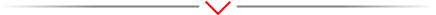# Black Sea Scientific Journal Of Academic ResearchOpinion Article - (2023) Volume 60, Issue 2

### MATHEMATICS: CONCEPTS, SUB-DISCIPLINES AND HISTORICAL DEVELOPMENT

Charles Ben*

Department of Mathematics, Jimma University, Jimma, Ethiopia

*Correspondence: Charles Ben, Department of Mathematics, Jimma University, Jimma, Ethiopia, Email:

Received: May 11, 2023, Manuscript No. BSSJAR-23-98635; Editor assigned: May 15, 2023, Pre QC No. BSSJAR-23-98635(PQ); Reviewed: May 29, 2023, QC No. BSSJAR-23-98635; Revised: Jun 05, 2023, Manuscript No. BSSJAR-23-98635(R); Published: Jun 12, 2023, DOI: 10.36962/GBSSJAR/60.2.008

The study of mathematics includes the study of quantities and their variations, forms and the spaces in which they are contained, equations and related structures, and number-related issues. These ideas are embodied in modern mathematics by the fundamental subfields of number theory, algebra, geometry and analysis, in that order. There is no widespread agreement among mathematicians over a standard definition for their academic field.

Concept of mathematics

The main focus of mathematics is the discovery of properties of abstract objects and their verification by pure reason. These entities are either abstractions from nature in modern mathematics or axioms items that are claimed to have certain properties. A proof is a set of logical rules applied to facts that have already been established. These results include axioms and theorems that have already been proven, as well as some fundamental characteristics that are regarded to be reliable starting points for the system being studied in the case of abstraction from nature.

The natural sciences, engineering, medicine, economics, computer science and social sciences all depend on mathematics. Despite the fact that mathematics is frequently used to represent phenomena, its core ideas are unrelated to scientific inquiry. It is common to classify certain branches of mathematics as applied mathematics, such as statistics and game theory, which are developed in parallel with their applications. Other subjects, which are referred to as pure mathematics because they were developed without any application whatsoever, usually later discover useful applications. For instance, the problem of integer factorization, which dates back to Euclid in 300 BC, had no practical use prior to its employment in the RSA cryptosystem, which is today widely used to secure computer networks.

Sub disciplines of mathematics

According to the Renaissance, mathematics was divided into two main areas: geometry, which is the study of shapes and arithmetic, which is the manipulation of numbers. At the time, it was difficult to discern between some pseudosciences like numerology and astrology and mathematics. Prior to the Renaissance, mathematics was divided into two main areas; geometry, which is the study of shapes and arithmetic, which is the manipulation of numbers. At the period, several pseudosciences, including numerology and astrology, were difficult to distinguish from mathematics.

Two more regions emerged during the Renaissance. Algebra, which is essentially the study and manipulation of formulas, was born out of mathematical notation. Calculus is the study of continuous functions, which simulate the frequently nonlinear correlations between the many values denoted by variables. Differential calculus and integral calculus are its two subfields. Math, geometry, algebra and calculus were divided into these four main categories until the end of the nineteenth century. At the time, mathematicians studied subjects that are now considered to be a component of physics, such as celestial mechanics and solid mechanics. Although combinatorics has been studied for the majority of recorded history, it wasn't until the seventeenth century that it was regarded as a distinct branch of mathematics.

The end of the nineteenth century they saw an explosion of new mathematical fields as a result of the fundamental crisis in mathematics and the following systematisation of the axiomatic method. According to the 2020 Mathematics Subject Classification, there are 63 first-level categories. Some of these fields relate to the prior division, such as number theory the modern name for advanced arithmetic and geometry. Many other first-level disciplines include "geometry" in their titles or are generally thought to be a component of geometry. Calculus and algebra are separated into several first-level topics but are not first-level domains. Other first-level disciplines, such mathematical foundations and logic, are modern inventions or subjects that were not previously categorised as mathematics.

Historical development

The idea of a proof and the mathematical rigour that goes along with it were first introduced in Greek mathematics, most notably in Euclid's Elements. Math had been basically divided between geometry and arithmetic, the manipulation of natural numbers and fractions, until the 16th and 17th centuries, when algebra and infinitesimal calculus were introduced as new disciplines. Since then, both have developed rapidly in lockstep as a result of the interaction between mathematical advances and scientific discoveries. The axiomatic method was systematised at the end of the 19th century as a result of the fundamental crisis in mathematics, which foreshadowed a fast expansion in the number of mathematical domains and their fields of application. There are more than 60 first-level study areas included in the current Mathematics Subject Classification.